# Speed Distance Time Speed Distance Time Calculations Bell

• Slides: 16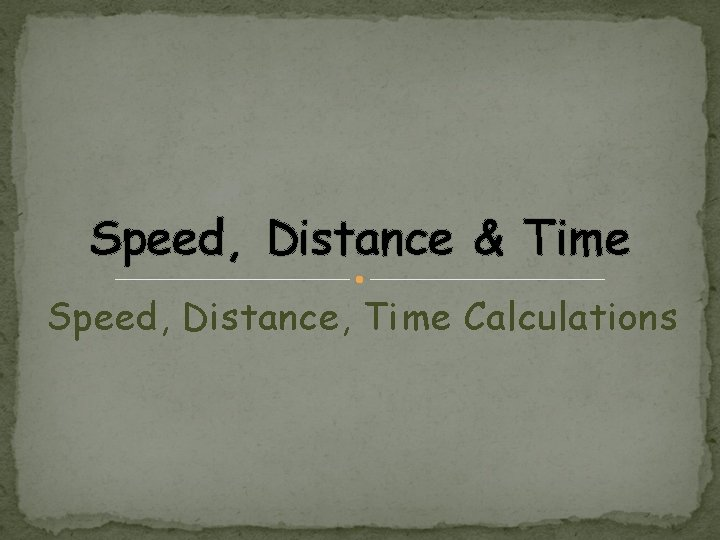Speed, Distance & Time Speed, Distance, Time CalculationsBell Work You Need: Colored Pencils Science Folder Science Notebook Everything else goes into the back. Start a new page in your notebook. Title it “Speed” What is speed? How do you think scientists define it? If you were to put speed into an equation what would it look like? Speed = ______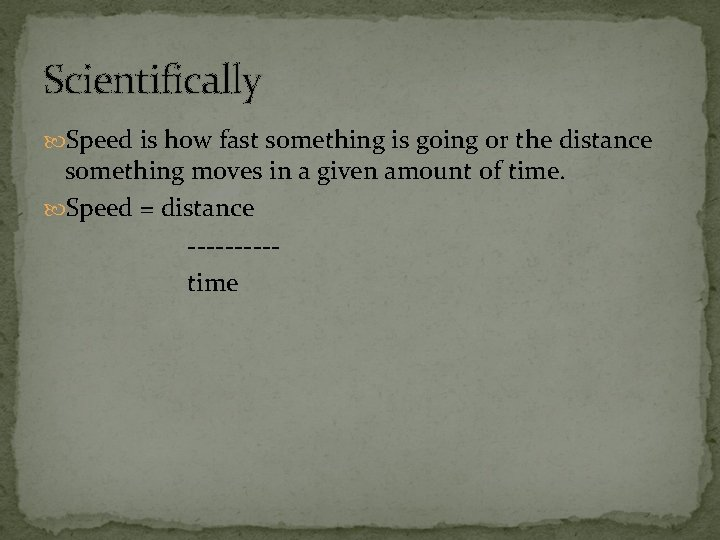Scientifically Speed is how fast something is going or the distance something moves in a given amount of time. Speed = distance -----time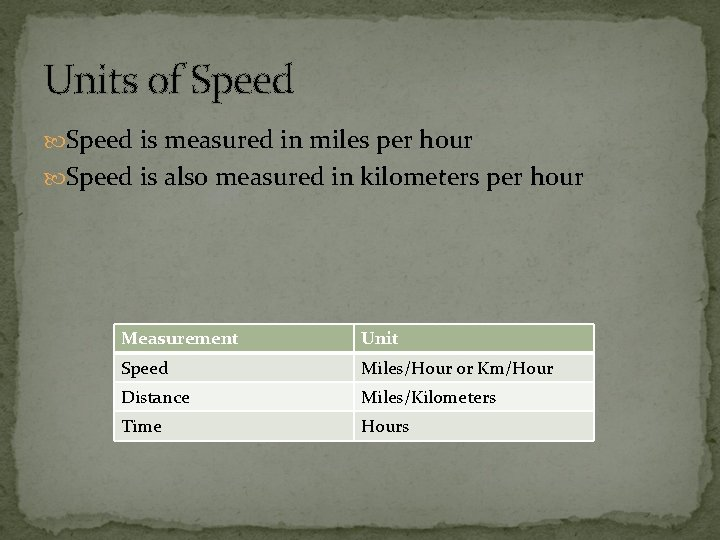Units of Speed is measured in miles per hour Speed is also measured in kilometers per hour Measurement Unit Speed Miles/Hour or Km/Hour Distance Miles/Kilometers Time Hours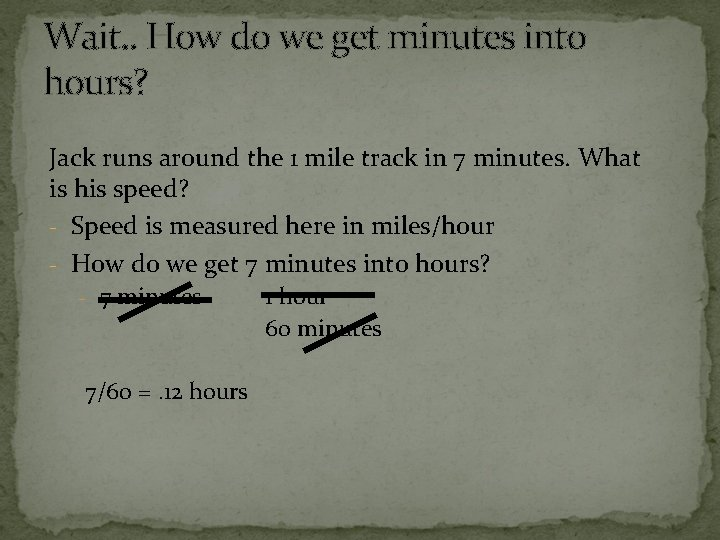Wait. . How do we get minutes into hours? Jack runs around the 1 mile track in 7 minutes. What is his speed? - Speed is measured here in miles/hour - How do we get 7 minutes into hours? - 7 minutes 7/60 =. 12 hours 1 hour 60 minutes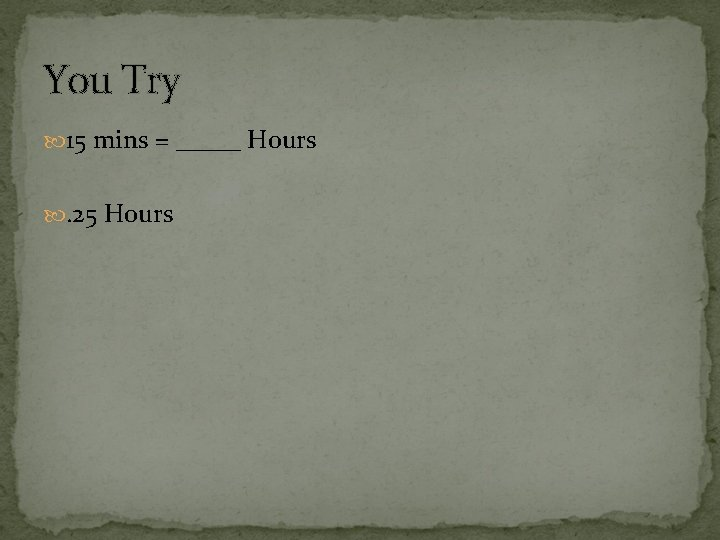You Try 15 mins = _____ Hours . 25 Hours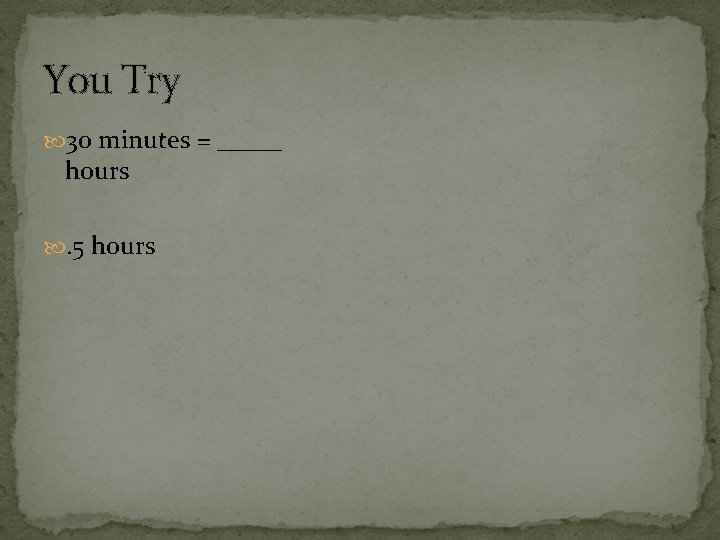You Try 30 minutes = _____ hours . 5 hours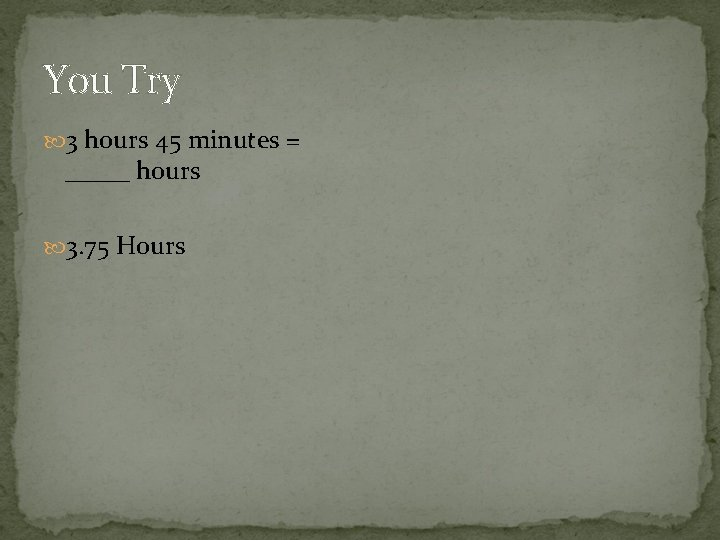You Try 3 hours 45 minutes = _____ hours 3. 75 Hours3 Formulas D S T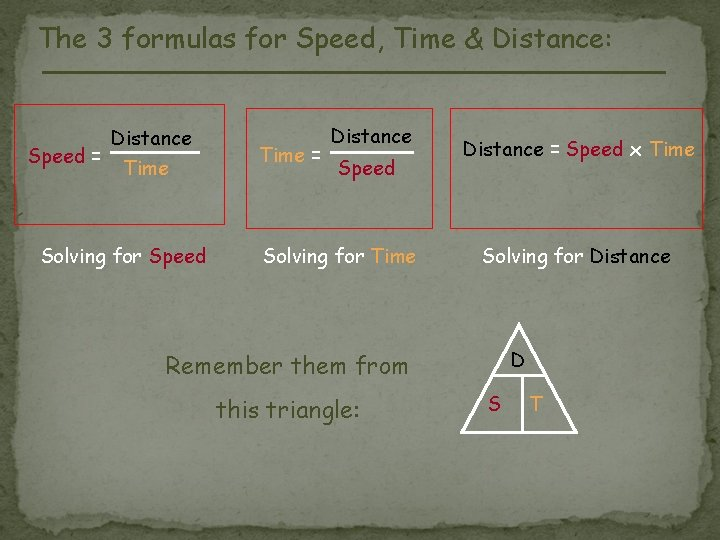The 3 formulas for Speed, Time & Distance: Speed = Distance Time Solving for Speed Time = Distance Speed Solving for Time Distance = Speed x Time Solving for Distance D Remember them from this triangle: S T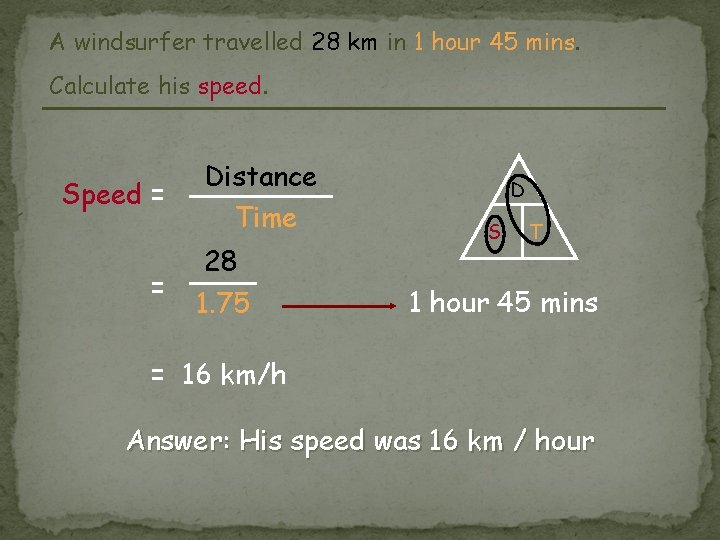A windsurfer travelled 28 km in 1 hour 45 mins. Calculate his speed. Speed = = Distance Time 28 1. 75 D S T 1 hour 45 mins = 16 km/h Answer: His speed was 16 km / hourA salesman travelled at an average speed of 50 km/h for 2 hours 30 mins. How far did he travel? Distance = Speed x Time = 50 x 2 • 5 D S T 2 hour 30 mins = 125 km Answer: He travelled 125 km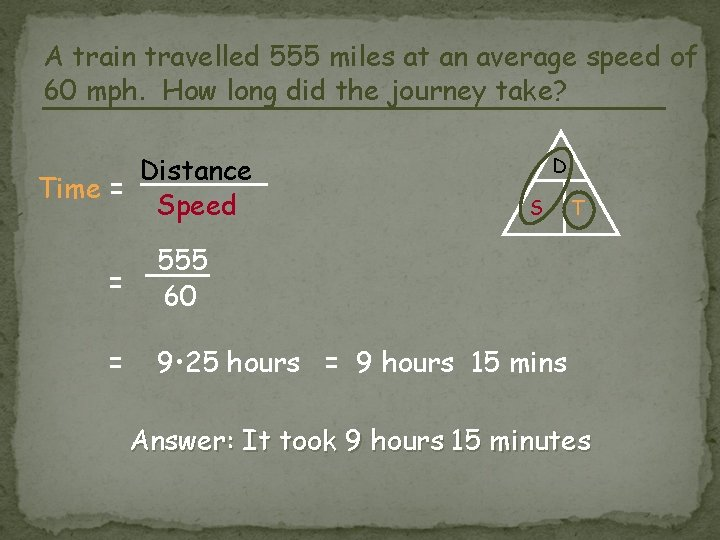A train travelled 555 miles at an average speed of 60 mph. How long did the journey take? Distance Time = Speed D S = 555 60 = 9 • 25 hours = 9 hours 15 mins T Answer: It took 9 hours 15 minutesTry It! Your dad traveled 75 miles in 2. 5 hours. What was his speed? 30 miles/hour Write your equation Change your units Fill in the info you know Solve Write proper units, circle your answer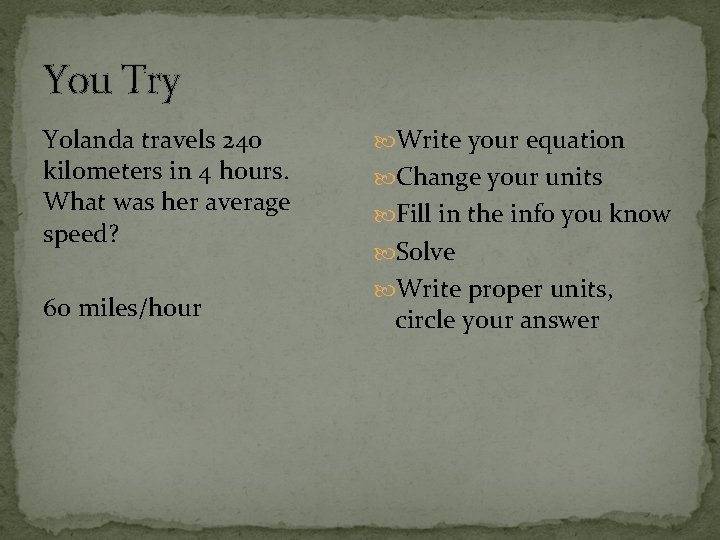You Try Yolanda travels 240 kilometers in 4 hours. What was her average speed? 60 miles/hour Write your equation Change your units Fill in the info you know Solve Write proper units, circle your answerYou Try! Mikes speed was 20 Write your equation km/hour. He traveled for Change your units 10 hours. How many Fill in the info you know kilometers did he drive? Solve Write proper units, 200 kilometers circle your answer